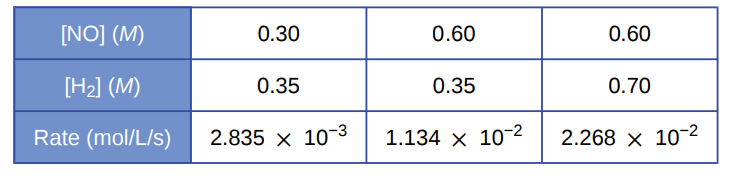# Problem: Hydrogen reacts with nitrogen monoxide to form dinitrogen monoxide (laughing gas) according to the equation:H2 (g) + 2NO(g) ⟶ N2O(g) + H2O(g) Determine the rate equation, the rate constant, and the orders with respect to each reactant from the following data:

###### FREE Expert Solution

Recall that the rate law only focuses on the reactant concentrations and has a general form of:

Calculate the order of the reaction with respect to NO.

 [NO] (M) 0.30 0.60 0.60 [H2] (M) 0.35 0.35 0.70 Rate (mol/L·s) 2.835x10-3 1.134x10-2 2.268x10-2

Calculate order with respect to NO:

93% (411 ratings)###### Problem Details

Hydrogen reacts with nitrogen monoxide to form dinitrogen monoxide (laughing gas) according to the equation:

H2 (g) + 2NO(g) ⟶ N2O(g) + H2O(g)

Determine the rate equation, the rate constant, and the orders with respect to each reactant from the following data:Frequently Asked Questions

What scientific concept do you need to know in order to solve this problem?

Our tutors have indicated that to solve this problem you will need to apply the Rate Law concept. You can view video lessons to learn Rate Law. Or if you need more Rate Law practice, you can also practice Rate Law practice problems.

What professor is this problem relevant for?

Based on our data, we think this problem is relevant for Professor Fowler's class at VALENCIA.

What textbook is this problem found in?

Our data indicates that this problem or a close variation was asked in Chemistry - OpenStax 2015th Edition. You can also practice Chemistry - OpenStax 2015th Edition practice problems.